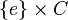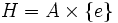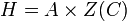# Center not is normality-preserving endomorphism-invariant

This article gives the statement, and possibly proof, of the fact that for a group, the subgroup obtained by applying a given subgroup-defining function (i.e., center) does not always satisfy a particular subgroup property (i.e., normality-preserving endomorphism-invariant subgroup)
View subgroup property satisfactions for subgroup-defining functions$|$ View subgroup property dissatisfactions for subgroup-defining functions

## Statement

It is possible to have a group$G$ such that the center$H = Z(G)$ is not normality-preserving endomorphism-invariant, i.e., there exists a normality-preserving endomorphism$\alpha$ of$G$ (i.e.,$\alpha$ sends normal subgroups to normal subgroups) but$\alpha(H)$ is not contained in$H$.

## Related facts

### Opposite facts

Below are some properties weaker than being normality-preserving endomorphism-invariant, that the center in fact satisfies:

Property Meaning Proof that center satisfies the property
strictly characteristic subgroup invariant under all surjective endomorphisms center is strictly characteristic
finite direct power-closed characteristic subgroup finite direct power of subgroup closed in corresponding power of whole group center is finite direct power-closed characteristic
direct projection-invariant subgroup invariant under projections to direct factors center is direct projection-invariant
characteristic subgroup invariant under all automorphisms center is characteristic

## Proof

### Generic example

Let$A$ be a nontrivial cyclic group and$C$ a centerless group containing a normal subgroup isomorphic to$A$, say$B$. Consider the direct product$G := A \times C$.

Clearly,$H := A \times \{ e \}$ (as an embedded direct factor) is the center of$A \times C$.

Now consider the endomorphism$\alpha$ of$G = A \times C$ which composes the projection from$G$ onto$A$ with an isomorphism from$A$ to$\{ e \} \times B$. Note that:

•$\alpha$ is normality-preserving: Its image,$\{ e \} \times B$ is a cyclic normal subgroup of a direct factor$\{ e \} \times C$. Since direct factor implies transitively normal and cyclic normal implies hereditarily normal, all subgroups of the image$\{ e \} \times B$ are normal in$G$. In particular, the image of any normal subgroup in$G$ is normal.
• The endomorphism does not send$H = A \times \{ e \}$ to itself: Rather, it gets mapped to$\{ e \} \times B$.

Find a group$A$ with an abelian normal subgroup$B$.

### Particular example

The above generic example can generate a particular example by setting$A$ as cyclic group:Z3 and$C$ as symmetric group:S3. The group$G$ is thus direct product of S3 and Z3.

### Modification of generic example for finite p-groups

The generic example outlined above does not work for finite$p$-groups, but the following slight modification does: instead of requiring$C$ to be a centerless group, simply require that$B$ not be contained in the center of$C$. In this case, the center of$G = A \times C$ becomes$H = A \times Z(C)$ where$Z(C)$ is the center of$C$. We construct the endomorphism in the same way (project to$A$, compose with isomorphism to$\{ e \} \times B$) and note that since$B$ is not contained in$Z(C)$, this endomorphism does not send$H$ to within itself.

### Particular examples for finite p-groups

•$p = 2$: We can take$A$ as cyclic group:Z4 and$C$ as dihedral group:D8 to get direct product of D8 and Z4. Alternatively, we can take$A$ as cyclic group:Z4 and$C$ as quaternion group to get direct product of Q8 and Z4.
•$p$ odd: Take$A$ as the cyclic group of prime-square order and$C$ as semidirect product of cyclic group of prime-square order and cyclic group of prime order.